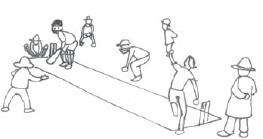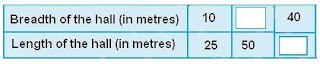## Things to remember..

When, we compared the two quantities in terms of ‘how many times’. This comparison is known as the Ratio. We denote ratio using symbol ‘:’ Consider the following example : DIsha’s weight is 15 kg and her father’s weight is 60 kg. How many times Father’s weight is of Isha’s weight? It is 4 times.
∴ The ratio of father’s weight to Disha’s weight = 60/15 = 4/1= 4:1
1. There are 20 girls and 15 boys in a class.
(a) What is the ratio of number of girls to the number of boys?
(b) What is the ratio of number of girls to the total number of students in the class?

 (a) The ratio of number of girls to the number of boys= 20/15 = 4/3 = 4 : 3 (b)To find out the ratio of number of girls to the total number of students in the class we have to first find the total numbers of students in the class i.e. 20+15 = 35 ∴ The ratio of number of girls the total number of students in the class= 20/35 = 4/7 = 4 : 7
2. Out of 30 students in a class, 6 like football, 12 like cricket and remaining like tennis. Find the ratio of
(a) Number of students liking football to number of students liking tennis.
(b) Number of students liking cricket to total number of students.(a) The ratio of Number of students liking football to number of students liking tennis= 6/(30-6-12) = 6/12 = 1 : 2 (b)The ratio of Number of students liking cricket to total number of students =12/ 30 = 2/5=2:5
3. See the figure and find the ratio of
(a) Number of triangles to the number of circles inside the rectangle.
(b) Number of squares to all the figures inside the rectangle.
(c) Number of circles to all the figures inside the rectangle.(a) The ratio of triangles to the number of circles inside the rectangle= 3/2 = 3 : 2 (b)The ratio of Number of squares to all the figures inside the rectangle. =2/ 7 = 2:7 (c)The ratio of Number of circles to all the figures inside the rectangle. =2/ 7 = 2:7

4. Distances travelled by Hamid and Akhtar in an hour are 9 km and 12 km. Find the ratio of speed of Hamid to the speed of Akhtar.
 The ratio of speed of Hamid to the speed of Akhtar= 9/12= 3/4 = 3:4
5. Fill in the following blanks : [Are these equivalent ratios?][All ratios represents 5/6]

6. Find the ratio of the following :
(a) 81 to 108
(b) 98 to 63
(c) 33 km to 121 km
(d) 30 minutes to 45 minutes

 (a) The ratio of 81 to 108= 81/108 = 3 : 4 (b)The ratio of 98 to 63 =14/ 9 = 14:9 (c)The ratio of 33 km to 121 km =33/ 121 = 3:11 (d)The ratio of 30 minutes to 45 minutes 30/45 = 2:3

7. Find the ratio of the following:
(a) 30 minutes to 1.5 hours
(b) 40 cm to 1.5 m
(c) 55 paise to Re 1
(d) 500 ml to 2 litres

 (a) The ratio of 30 minutes to 1.5 hours(1.5 hours=1.5×60 Minutes) 30/90 = 30: 90 = 1:3 (b)The ratio of 40 cm to 1.5 m (1.5 m = 1.5 ×100 cm) =40/ 150 = 4:15 (c)The ratio of 55 paise to Re 1 (Re 1=100 paise) =55/ 100 = 11:20 (d)The ratio of 500 ml to 2 litres (2 liitres= 2× 1000 ml) = 500/2000=1:4

8. In a year, Seema earns Rs 1,50,000 and saves Rs 50,000. Find the ratio of
(a) Money that Seema earns to the money she saves.
(b) Money that she saves to the money she spends.
 (a) The ratio of Money that Seema earns to the money she saves. = 1,50,000/50,000= 3/1 = 3:1 (b)The money she spends=1,50,000-50,000 = 1,00,000 (c)The ratio of Money that she saves to the money she spends= 50,000/1,00,000= 1/2 = 1:2
9. There are 102 teachers in a school of 3300 students. Find the ratio of the number of teachers to the number of students.
 The ratio of the number of teachers to the number of students = 102/3300= 17/550 = 17 : 550
10. In a college, out of 4320 students, 2300 are girls. Find the ratio of
(a) Number of girls to the total number of students.
(b) Number of boys to the number of girls.
(c) Number of boys to the total number of students.

 (a) The ratio of Number of girls to the total number of students = 2300/4320= 115/216 = 115 : 216 (b) The Numbers of boys= 4320 - 2300 = 2020 The ratio of Number of boys to the number of girls = 2020/2300 = 101/115 = 101:115 (c) The ratio of Number of boys to the total number of students= 2020/4320= 101/216 = 101:216
11. Out of 1800 students in a school, 750 opted basketball, 800 opted cricket and remaining opted table tennis. If a student can opt only one game, find the ratio of

(a) Number of students who opted basketball to the number of students who opted table tennis.
(b) Number of students who opted cricket to the number of students opting basketball.
(c) Number of students who opted basketball to the total number of students.

 (a) The number of students who opted table tennis = 1800 - 750 - 800 = 250 The ratio of Number of students who opted basketball to the number of students who opted table tennis= 750/250 = 3/1 = 3:1 (b) The ratio of Number of students who opted cricket to the number of students opting basketball. = 800/750 = 16/15 = 16 : 15 (c) The ratio of Number of students who opted basketball to the total number of students = 750/1800 = 5/12 = 5:12
12. Cost of a dozen pens is Rs 180 and cost of 8 ball pens is Rs 56. Find the ratio of the cost of a pen to the cost of a ball pen.

 The Cost of a dozen pens is = Rs 180 and ∴ cost of 1 pen is = 180/12 = Rs 15 The cost of 8 ball pens is = Rs 56 ∴ cost of 1 ball pen is = 56/8 = Rs 7 The ratio of cost of a pen to the cost of a ball pen = 15/7 = 15 : 7

13. Consider the statement: Ratio of breadth and length of a hall is 2 : 5. Complete the following table that shows some possible breadths and lengths of the hall.Add caption

All possible  breadths and lengths of the hall are simply parts of the equivalent ratio of 2:5

14. Divide 20 pens between Sheela and Sangeeta in the ratio of 3 : 2.

 The sum of the parts (ratios) = 3+2 = 5 The numbers of pens, Sheela will get = (3/5)×20 = 12 The numbers of pens, Sangeeta will get = (2/5)×20 = 8
15. Mother wants to divide Rs 36 between her daughters Shreya and Bhoomika in the ratio of their ages. If age of Shreya is 15 years and age of Bhoomika is 12 years, find how much Shreya and Bhoomika will get.The ratio of age of Shreya to the age of Bhoomika = 15 /12 = 5: 4 The sum of the parts (ratios) = 5+4 = 9 The Rs Shreya will get = (5/9)×36 = Rs 20 The Rs Shreya will get = (4/9)×36 = Rs 16
16. Present age of father is 42 years and that of his son is 14 years. Find the ratio of
(a) Present age of father to the present age of son.
(b) Age of the father to the age of son, when son was 12 years old.
(c) Age of father after 10 years to the age of son after 10 years.
(d) Age of father to the age of son when father was 30 years old. Answer:
 (a) The ratio of Present age of father to the present age of son = 42 /14 = 3:1 (b) The age of the father, When son was 12 = 42-(14-12) = 40 The ratio of the age of the father to the age of son, when son was 12 years old = 40/12 = 10/3= 10:3 (c) The ratio of the age of father after 10 years to the age of son after 10 years = (42+10)/(14+10) = 52/24=13/6 = 13 : 6 (d) The ratio of the age of father to the age of son when father was 30 years old. = 30/[14-(42-30)] = 30/(14 -12)= 30/2 = 15/1 = 15 : 1

1.2.3.